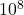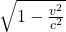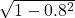## An astronaut named Speedo takes a trip to Sirius, which is located a distance of 8 lightyears from the Earth. If the spaceship moves at a co

Question

An astronaut named Speedo takes a trip to Sirius, which is located a distance of 8 lightyears from the Earth. If the spaceship moves at a constant speed of 0.8 c, how long does it take for Speedo to reach Sirius? If the trip of Speedo is observed with a very powerful telescope by his twin brother Goslo in Mission Control on the Earth, at what time will Goslo see that Speedo has arrived at Sirius? Right after reaching the Sirius, Speedo immediately turns around and comes back home. When does he arrive according to Speedo himself? To his twin brother Goslo? (The Twin Paradox)

in progress 0
5 months 2021-08-29T12:54:33+00:00 1 Answers 9 views 0

a. 10 years

b. 6 years

c. 12 years behind

Explanation:

speed of light c =  3xm/s

distance of sirius from earth = 8 lightyears = 8 x c x 60 x 60 x 24 x 365 = 252288000c meters

speed of spaceship = 0.8c

time taken to reach sirius = distance covered divided by speed

t = d/s = 252288000c/0.8c = 315360000 sec

1 year = 60 x 60 x 24 x 365 sec = 31536000 sec

315360000 sec = 315360000/31536000 = 10 yrs

the time as observed by Goslo will be shorter than the real time as measured within the ship due to relativistic effects. using the relativistic relation for time dilation we have

t’ = tsince v = 0.8c

v/c = 0.8

where t’ is the relativistic time as observed by Goslo,

t is the time within the ship

t’ = 10 x= 6 yrs

If speedo turns around immediately after reaching  sirius, his total distance will be 252288000c x 2 = 50457600c meter

time taken = 50457600c/0.8 = 630720000 sec = 20 yrs

time within the ship will be calculated from time dilation

t’ = tt’ = 20 x= 378432000 sec = 12 years behind